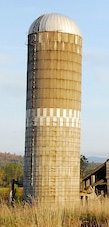SEARCH HOMEMath Central Quandaries & QueriesQuestion from Monalizsha: What is the volume and the surface area of the hemisphere and cylinder (both are together) The height is 12cm of the cylinder and the diameter is 6cmHi Monalizsha,

Are the hemisphere and cylinder together like a silo?If so you can treat the two shapes independently. The volume of the silo is the volume of the hemisphere plus the volume of the cylinder and likewise for the surface area.

The volume of a hemisphere can be found here and volume of a cylinder can be found here

The surface area of a hemisphere can be found here and the and the surface area of a cylinder can be found here.

Let us know if you need more assistance,
PennyMath Central is supported by the University of Regina and The Pacific Institute for the Mathematical Sciences.